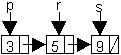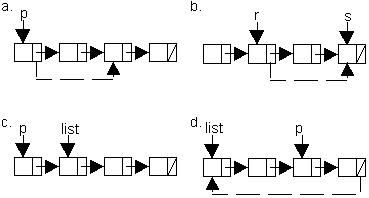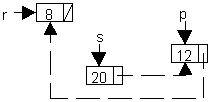Exercises
Pointers #2

Use the declaration below to answer the following questions.

```    node *p, *r, *s;    p = NULL;    s = new node;    r = s; ```

1. Draw a diagram that illustrates each of the three ways to assign a pointer.

2. Show what each of the following statements do to its pointer diagram.
 `p = p -> next;` `r = p;` `s = p -> next;` `p -> info = r -> info;` `p -> info = r -> next -> info;` `s -> next = p;`3. Write one statement using the `->` notation, to make the change indicated by the dotted line.4. ``` node *p, *r,*s; p = new node; s = new node; r = new node; p -> next = r; r -> next = s; s -> next = p; s -> info = 3; p ->next -> next -> next -> info = 2; s -> next -> next -> info = 1; p = s -> next; cout << p -> info << " " << s -> info << " " << r -> info; ```

5. ```node *p,*s; s = new node; p = new node; p -> info = 7; s->info = 8; s -> next = p; s -> next -> info = 5; s -> next -> next = p; p -> next -> info = 4; s ->info = p -> next ->info; cout << p -> info << " " << s -> next -> next -> info << " " << q -> info; ```

6. Write only 9 statements to form this diagram.Continue to:  Unit 5 / Prev / Next